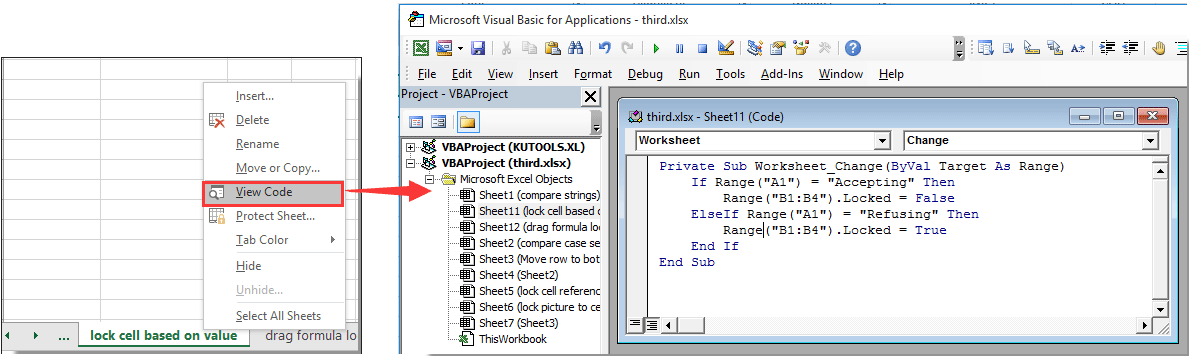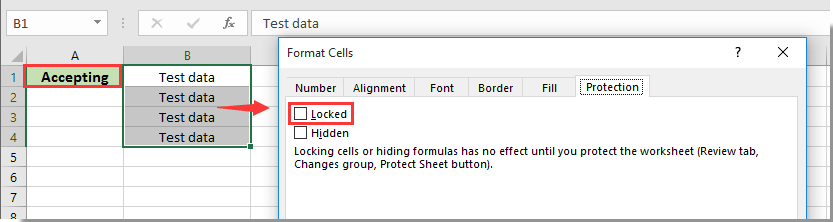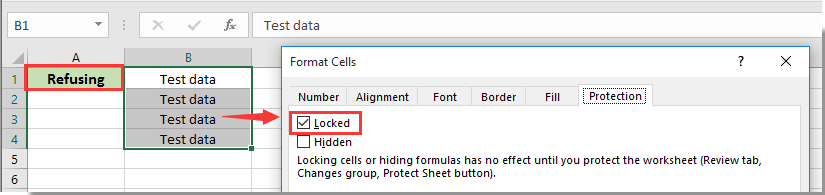Note: The other languages of the website are Google-translated. Back to English

## 如何根据Excel中另一个单元格中的值锁定或解锁单元格？

#### 使用VBA代码根据另一个单元格中的值锁定或解锁单元格

1.右键单击工作表选项卡（带有您需要根据另一个单元格中的值锁定或解锁单元格的工作表），然后单击 查看代码 从右键单击菜单中。

2.然后将以下VBA代码复制并粘贴到“代码”窗口中。

VBA代码：根据另一个单元格中的值锁定或解锁单元格

``````Private Sub Worksheet_Change(ByVal Target As Range)
If Range("A1") = "Accepting" Then
Range("B1:B4").Locked = False
ElseIf Range("A1") = "Refusing" Then
Range("B1:B4").Locked = True
End If
End Sub``````3。 按 其他 + Q 同时关闭按键 Microsoft Visual Basic应用程序 窗口。### 最佳办公效率工具

#### Kutools for Excel解决了您的大多数问题，并使您的生产率提高了80％

• 重用: 快速插入 复杂的公式，图表 以及您以前使用过的任何东西； 加密单元 带密码 创建邮件列表 并发送电子邮件...
• 超级公式栏 （轻松编辑多行文本和公式）； 阅读版式 （轻松读取和编辑大量单元格）； 粘贴到过滤范围...
• 合并单元格/行/列 不会丢失数据； 拆分单元格内容； 合并重复的行/列...防止细胞重复； 比较范围...
• 选择重复或唯一 行; 选择空白行 （所有单元格都是空的）； 超级查找和模糊查找 在许多工作簿中； 随机选择...
• 确切的副本 多个单元格，无需更改公式参考； 自动创建参考 到多张纸； 插入项目符号，复选框等...
• 提取文字，添加文本，按位置删除， 删除空间; 创建和打印分页小计； 在单元格内容和注释之间转换...
• 超级滤镜 （将过滤方案保存并应用于其他工作表）； 高级排序 按月/周/日，频率及更多； 特殊过滤器 用粗体，斜体...
• 结合工作簿和工作表; 根据关键列合并表； 将数据分割成多个工作表; 批量转换xls，xlsx和PDF...
• 超过300种强大功能. 支持 Office / Excel 2007-2021 和 365。支持所有语言。 在您的企业或组织中轻松部署。 完整功能 30 天免费试用。 60 天退款保证。#### Office选项卡为Office带来了选项卡式界面，使您的工作更加轻松

• 在Word，Excel，PowerPoint中启用选项卡式编辑和阅读，发布者，Access，Visio和Project。
• 在同一窗口的新选项卡中而不是在新窗口中打开并创建多个文档。
• 每天将您的工作效率提高50％，并减少数百次鼠标单击！Range("A1").Locked = False
Range("B1:B4").Locked = False

END SUB
Private Sub Worksheet_SelectionChange（作为范围的ByVal目标）

Application.EnableEvents = False

Application.EnableEvents = True

ElseIf ActiveSheet.ProtectContents _

xRgA.选择

Application.EnableEvents = True
END SUB

Private Sub Workbook_Open() '这进入“ThisWorkbook”

END SUB

Range("A1").Locked = False
Range("B1:B4").Locked = False

END SUB
Private Sub Worksheet_SelectionChange（作为范围的ByVal目标）

Application.EnableEvents = False

Application.EnableEvents = True

ElseIf ActiveSheet.ProtectContents _

xRgA.选择

Application.EnableEvents = True
END SUB

Range("D40:E40").Locked = False
ElseIf Range("A40") = "" 那么
Range("D40:E40").Locked = True

END SUB

Range("B1").Locked = True
Range("B2").Locked = True
Range("B3").Locked = True

END SUB

Мне тоже необходимо заблокировать значение, но только в одной ячейке в зависимости от значения в другой, я попробовала использовать код в комментарии выше, но он не работает(оставила только один параметр для блокировки), но он у меня не работает - при этом нет сообщения об ошибке, ячейка, которая должна быть заблокирована - просто не блокируется, остается активной。 В чем может быть причина？

Вот код, который я использовала:

END SUB

PreVal = 范围（“A1”）
NextVal = 范围（“A1”）
END SUB

If (Target.Count = 1) And (Target.Address = "\$A\$1") Then
NextVal = 范围（“A1”）

END SUB
Private Sub Worksheet_SelectionChange（作为范围的ByVal目标）

PreVal = 范围（“A1”）

Application.EnableEvents = False

Application.EnableEvents = True

END SUB

If Range("Cells(D, i)") = "Loan" Then
Range("Cells(V, i):Cells(X, i)").Locked = True
ElseIf Range("Cells(D, i)") = "Savings" Then
Range("Cells(Q, i):Cells(U, i)").Locked = True
Range("Cells(W, i):Cells(X, i)").Locked = True
ElseIf Range("Cells(D, i)") = "ShareCap" Then
Range("Cells(Q, i):Cells(U, i)").Locked = True
Range("Cells(V, i)").Locked = True

END SUB

1. 发票模板（发票）- 只是每周发送给我的代理的通用发票。

2.准确的数据表（数据表）-发票可以读取公司名称地址等，因此如果有任何更改，发票将自动更新。

3. 确切地说是日历选项卡（Calendar 2018） - 在发票模板中引用，并将相应的日期和发票编号放在实际发票上。

Sheets("数据表").Protect
ElseIf xRg.Value = "Active" Then
Sheets("数据表").Unprotect

END SUB

Range("S:S").Locked = True
ElseIf Range("K:K") = "SLOW MOVING" Then
Range("S:S").Locked = True
ElseIf Range("K:K") = "NORMAL" Then
Range("S:S").Locked = False
ElseIf Range("K:K") = "SHORTAGE" Then
Range("S:S").Locked = False

END SUB

If Range("A:A") = "SLOW MOVING" Then
Range("B:B").Locked = True
ElseIf Range("A:A") = "OVER STOCK" Then
Range("B:B").Locked = True
ElseIf Range("A:A") = "NORMAL" Then
Range("B:B").Locked = False

END SUB

If Range("A3:A37").Value <> "" Then
Range("B3:B37").Locked = True
ElseIf Range("A3:A37") = "" 那么
Range("B3:B37").Locked = False

If Range("B3:B37").Value <> "" Then
Range("A3:A37").Locked = True
ElseIf Range("B3:B37") = "" 那么
Range("A3:A37").Locked = False

END SUB

Hi

hi

A2 用于 D2 到 F2

Range("C94:F104").Locked = False

ElseIf Range("D90") = "No" Then

Range("C94:F104").Locked = True

END SUB

D90 不在范围内。 在 C4 上方 94 行

0   产品特性

## 关注我们

Microsoft和Office徽标是Microsoft Corporation在美国和/或其他国家的商标或注册商标。